• 异常检测之孤立森林（isolate forest）
千次阅读
2021-12-01 16:25:26

大家好，我是菜菜卷！今天开始陆续和大家分享一些关于异常检测入门相关的实战项目（包括使用sklearn实现一些简单的机器学习模型或者使用pytorch实现简单的深度学习模型）
今天我们使用的模型是集成于sklearn内部实现的孤立森林算法。

# 什么是孤立森林（isolate forest）？

孤立森林是一种传统的异常检测机器学习算法，他属于无监督学习的boost 树模型，对于每棵子树来说，针对数据集的不同特征值，会随机挑选特征值取值范围内的一个随机值，然后将所有数据按照该特征的这个随机值划分为两部分（根据该特征值大于或者小于该随机值），这样我们就可以逐渐将所有的数据分开，因为异常数据通常来说所占的比例都较少，所以一般使用较少的属性就可以将他们从正常数据中区分开来，因此平均路径长度便可以作为是否是异常值的衡量指标（异常数据的平均路径长度会比正常数据更短）

# 所用数据集

数据集获取地址：KDD1999Data
前往以上的地址，下载kddcup.data.gz即可。
该数据集是由若干正常和异常的数据分类，有41和特征和1个标签构成，因为后面我们只想对网页攻击方向的数据进行分析，所以要强调一下，第三个特征是表示异常的方向（即是哪种方面的异常，比如http还是其他）

# 项目所用环境

numpy 1.15.3
pandas 0.23.4
scikit-learn 0.19.1
matplotlib 2.2.2


大家可以使用pip或者conda自行安装项目环境，为保证顺利无bug复现结果，建议使用所示版本的包

# 项目实战

## 1、包的导入

首先我们先导入实验所需的第三方包

import time
import numpy as np
import pandas as pd
import matplotlib.pyplot as plt
from sklearn.ensemble import IsolationForest
from sklearn.model_selection import train_test_split
from sklearn.preprocessing import LabelEncoder
from sklearn.metrics import roc_auc_score


其中time可以用来记录时间和程序运行时间；numpy可以高效做矩阵运算，在数据处理中有广泛的运用；pandas可以快速方便的读入和处理数据的格式；matplotlib用于部分实验结果的可视化；sklearn是一个非常丰富的机器学习库，内部集成了基本的机器学习方法、数据集处理方法、模型衡量指标等功能。

## 2.使用pandas读入和处理数据

首先我们使用以下代码来读入数据：

data_path = r'dataset/kddcup.data.corrected'
columns = [str(i) for i in range(1, 42)]
columns.append('label')
df = pd.read_csv(data_path, sep=',', names=columns, index_col=None)


其中columns是我们给每个特征的命名，具体是什么都可以，只需要后续操作特征的时候可以对应上最开始的命名就可以了，这里我就简单的将最开始的41列命名为1-41的str类型数字，最后一列（第42列）命名为'label'names=columns表示我们使用自定义的columns来命名数据中的42个列。
下面我们使用print(df.shape)看一下数据的大小，结果如下所示：

(4898431, 42)


说明我们的数据集有接近500w的数据，其中每个数据有42个属性（其中前41个位特征值，第42个是标签label）。
接下来我们简单的处理一下数据，假设目前我们仅关心与http相关的数据是否正常（第3列特征描述数据的分类），我们使用如下代码来挑选出数据集中所有和http相关的数据：

df = df[df['3'] == 'http']
df = df.drop('3', axis=1)
columns.remove('3')


其中df['3'] == 'http'用来判断数据df的第三个属性是否是http，是的话就是True，否的话就是False，所以df = df[df['3'] == 'http']实现的功能就是找到所有第三列属性是http的数据，因为在找到后，我们的第三列属性就无用了（因为都是http了），所以我们用df = df.drop('3', axis=1)将第三列属性去掉，下面我们再使用print(df.shape)看看数据的shape，结果如下所示：

(623091, 41)


我们可以发现，数据从原来的接近500w变成了现在的62w个，属性值也从42变成了41，说明我们以上操作成功了！

然后我们运行print(df['label'].value_counts())来观察一下label的取值，结果如下所示：

normal.       619046
back.           2203
neptune.        1801
portsweep.        16
ipsweep.          13
satan.             7
phf.               4
nmap.              1
Name: label, dtype: int64


从结果中我们可以发现，大多数数据都是正常的（normal.）,但是也有一些其他异常的数据，我们本次的核心任务就是使用孤立森林模型来完成对数据正常与否的检测。

我们希望更详细的观察一些数据的情况，使用print(df.head(5))来显示前五个数据的详细信息：

  1    2   4    5      6  7  8  9  ...   35    36   37   38   39   40   41    label
0  0  tcp  SF  215  45076  0  0  0  ...  0.0  0.00  0.0  0.0  0.0  0.0  0.0  normal.
1  0  tcp  SF  162   4528  0  0  0  ...  0.0  1.00  0.0  0.0  0.0  0.0  0.0  normal.
2  0  tcp  SF  236   1228  0  0  0  ...  0.0  0.50  0.0  0.0  0.0  0.0  0.0  normal.
3  0  tcp  SF  233   2032  0  0  0  ...  0.0  0.33  0.0  0.0  0.0  0.0  0.0  normal.
4  0  tcp  SF  239    486  0  0  0  ...  0.0  0.25  0.0  0.0  0.0  0.0  0.0  normal.


通过结果我们可以发现，不同属性对应的类型也是不一样的，对于1,5,6这种int类型的或者35,36这种float类型的属性可以不做处理直接使用，但是对于24这种object类型的属性的话，是不能直接使用的。需要对其进行编码，我们使用下面的方式对其编码：

for col in df.columns:
if df[col].dtype == 'object':
encoded = LabelEncoder()
encoded.fit(df[col])
df[col] = encoded.transform(df[col])


我们遍历数据中的所有特征，如果其类型是object的话，我们要使用sklearn中的LabelEncoder()来对其编码，编码后我们使用print(df.head(5))看一下编码后的效果：

   1  2  4    5      6  7  8  9  ...   35    36   37   38   39   40   41  label
0  0  0  9  215  45076  0  0  0  ...  0.0  0.00  0.0  0.0  0.0  0.0  0.0      4
1  0  0  9  162   4528  0  0  0  ...  0.0  1.00  0.0  0.0  0.0  0.0  0.0      4
2  0  0  9  236   1228  0  0  0  ...  0.0  0.50  0.0  0.0  0.0  0.0  0.0      4
3  0  0  9  233   2032  0  0  0  ...  0.0  0.33  0.0  0.0  0.0  0.0  0.0      4
4  0  0  9  239    486  0  0  0  ...  0.0  0.25  0.0  0.0  0.0  0.0  0.0      4

[5 rows x 41 columns]


从上述结果展示中我们看到：之前第2列和第4列都已经成功变成了非object类的属性了（这种方式其实在深度学习领域有个专门的名字叫OneHot编码，感兴趣的同学可以深入了解一下），经过以上处理后，我们基本上已经得到了想要的数据集了，下面我们将详细介绍一下如何切分数据集。

## 3.划分数据集

除了要通过数据得到模型以外，我们还需要测试一下他的泛化能力（也就是在未见过的数据中是否也有同样好的效果），因此我们要将得到的数据划分为训练集、验证集和测试集。

因为我们不知道原数据集中的数据是否是有顺序的，所以为了避免异常数据集中出现在某一集合中（训练集或者验证集或者测试集），所以我们使用如下代码随机打乱数据的顺序：

for _ in range(3):
df = df.iloc[np.random.permutation(len(df))]


在获得乱序的数据集后，我们要进行数据的切分，此处我们使用前50w的数据来作为训练集和测试集，使用50w之后的数据作为验证集，其代码如下：

df2 = df[:500000]
labels = df2['label']
df_validate = df[500000:]

x_val, y_val = df_validate, df_validate['label']
x_train, x_test, y_train, y_test = train_test_split(df2, labels, test_size=0.2, random_state=42)


其中train_test_splitsklearn内置的一种划分训练集和测试集的方式，参数test_size=0.2表示测试集所占的比例（我们此时使用前50w数据的20%作为测试集）。
下面我们看一下划分的结果：

print('the shape of x_train, x_test, x_val is {}, {} and {}'.format(x_train.shape, x_test.shape, x_val.shape))


其结果如下：

the shape of x_train, x_test, x_val is (400000, 41), (100000, 41) and (123091, 41)


我们可以看到，其结果如我们所料，这样数据集就划分好了！！

## 4、树模型的构建和训练

首先我们使用以下代码来构建并训练孤立森林模型：

isolation_forest = IsolationForest(n_estimators=100, max_samples=256, contamination=0.1, random_state=42)
print("---------------------start training-------------------")
start_time = time.time()
isolation_forest.fit(x_train)
print('the training costs {}s'.format(time.time() - start_time))
print("---------------------end training-------------------")


其中time.time()只是用来记录开始训练和训练结束的时间，从而得到训练的耗时。

## 测试模型泛化能力

模型只在训练集上表现好是远远不够的，因为可能会出现过拟合的情况，所以还需要在训练时未见过的数据上测试其泛化能力（这也是我们划分训练集验证集和测试集的原因），首先我们使用训练好的孤立森林模型对验证集数据做预测：

anomaly_scores = isolation_forest.decision_function(x_val)


anomaly_scores即为预测结果，其是一个一维的向量，存储了每个数据的预测分数，我们先使用以下代码可视化看一下其分数的分布情况：

plt.figure(figsize=(15, 10))
plt.hist(anomaly_scores, bins=100)
plt.xlabel('Average Path Lengths', fontsize=14)
plt.xlabel('Number of Data Points', fontsize=14)
plt.show()


其结果如下所示：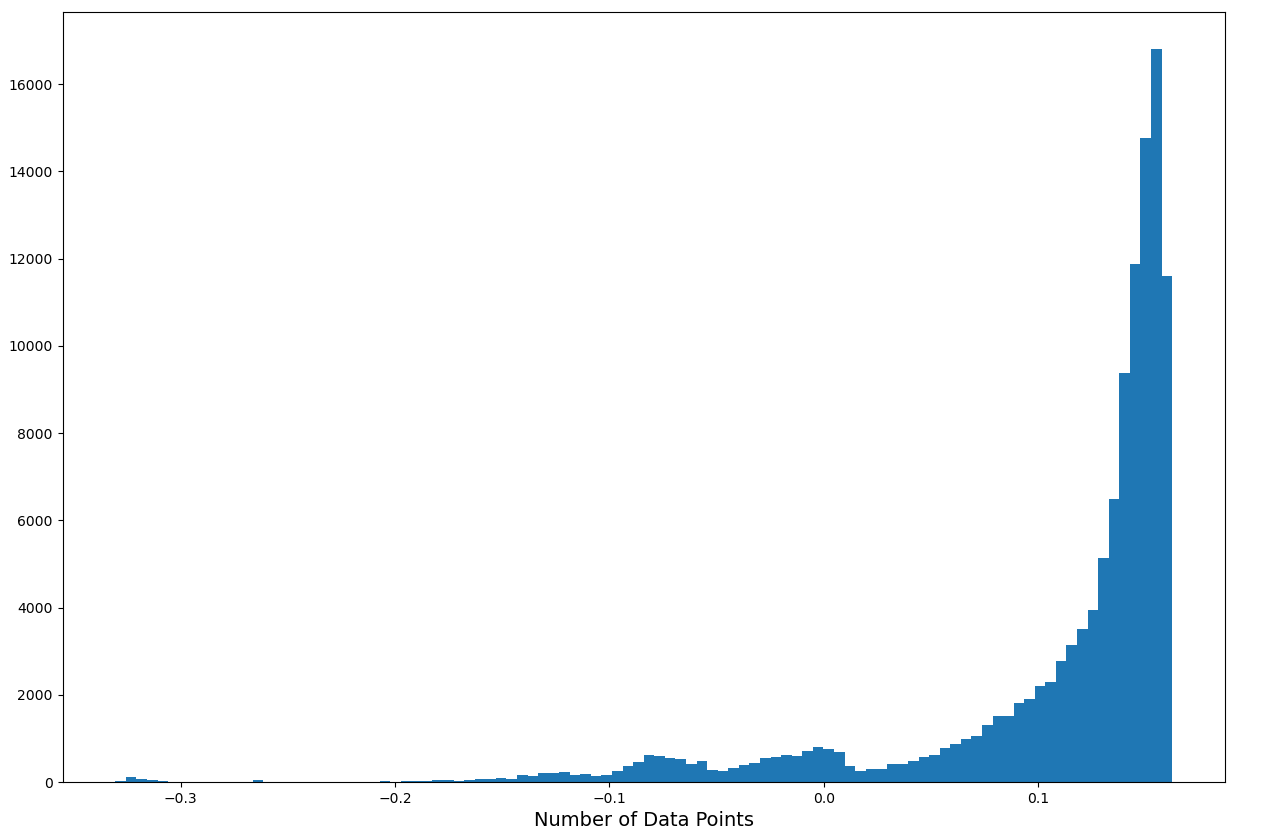我们可以看到，大多数数据的最短路径都是较长的，但是也有个别的数据路径非常短（例如小于-0.19），那么我们就有很大的把握认为路径较短的数据是异常数据，假设我们认定路径长度小于-0.19的是异常数据，那么我们使用训练好的模型看看AUC是多少：

anomalies = anomaly_scores > -0.19
matches = y_val == list(encoded.classes_).index('normal.')
# print('the shape of anomalies and matches are', anomalies.shape, matches.shape)
auc = roc_auc_score(anomalies, matches)
print("AUC:{:.2%}".format(auc))


其中anomaly_scores > -0.19会生成一个list，其shapeanomaly_scores是相同的，每个元素都是True或者False，表示该数据的分数是否大于-0.19，所以anomalies存储的是模型的预测值；

我们在处理数据的时候曾经用LabelEncoder()来将object类的属性转换为onehot编码的值，因此此处的encoded实际上就是最后一个object类的属性（即我们自定义为'label'的最后一列特征）,所以list(encoded.classes_).index('normal.')的功能就是获得'label'列中原来为normal.的数据编码后的onehot编码,所以y_val == list(encoded.classes_).index('normal.')也可以获得一个和y_valshape相同的值为True或者Falselist，所以matches存储的是真实值。

之后我们再使用sklearn自带的roc_auc_score来计算该模型在验证集上的auc，其结果如下所示：

AUC:98.36%


可见我们训练好的孤立森林确实可以实现异常数据的检测，而且其效果和泛化能力还挺不错。

这些大致就是异常检测中传统机器学习算法孤立森林相关的实战内容了，其完整代码如下：

import time
import numpy as np
import pandas as pd
import matplotlib.pyplot as plt
from sklearn.ensemble import IsolationForest
from sklearn.model_selection import train_test_split
from sklearn.preprocessing import LabelEncoder
from sklearn.metrics import roc_auc_score

if __name__ == '__main__':
data_path = r'dataset/kddcup.data.corrected'
columns = [str(i) for i in range(1, 42)]
columns.append('label')
df = pd.read_csv(data_path, sep=',', names=columns, index_col=None)
# print(df.shape)
df = df[df['3'] == 'http']
df = df.drop('3', axis=1)
columns.remove('3')
# print(columns)
# print(df.shape)
# print(df['label'].value_counts())
for col in df.columns:
if df[col].dtype == 'object':
encoded = LabelEncoder()
encoded.fit(df[col])
df[col] = encoded.transform(df[col])
for _ in range(3):
df = df.iloc[np.random.permutation(len(df))]
df2 = df[:500000]
labels = df2['label']
df_validate = df[500000:]

x_val, y_val = df_validate, df_validate['label']
x_train, x_test, y_train, y_test = train_test_split(df2, labels, test_size=0.2, random_state=42)
#
# print('the shape of x_train, x_test, x_val is {}, {} and {}'.format(x_train.shape, x_test.shape, x_val.shape))

isolation_forest = IsolationForest(n_estimators=100, max_samples=256, contamination=0.1, random_state=42)
print("---------------------start training-------------------")
start_time = time.time()
isolation_forest.fit(x_train)
print('the training costs {}s'.format(time.time() - start_time))
print("---------------------end training-------------------")

anomaly_scores = isolation_forest.decision_function(x_val) # 一个一维的list，存储了每个数据的路径长度
# plt.figure(figsize=(15, 10))
# plt.hist(anomaly_scores, bins=100)
# plt.xlabel('Average Path Lengths', fontsize=14)
# plt.xlabel('Number of Data Points', fontsize=14)
# plt.show()

anomalies = anomaly_scores > -0.19
matches = y_val == list(encoded.classes_).index('normal.')
# print('the shape of anomalies and matches are', anomalies.shape, matches.shape)
auc = roc_auc_score(anomalies, matches)
print("AUC:{:.2%}".format(auc))


我是菜菜卷，我们下篇再见！机器学习 sklearn 异常检测
更多相关内容
• 孤立森林

孤立森林，不再描述正常的样本点，而是要孤立异常点。

核心思想：异常的点通常是孤立的，例如采用二叉树对数据进行切分，数据点在二叉树中所处的深度反应了该条数据的“疏离”程度，异常点只需较少的切分即可划分出来。

异常的两个特点：异常数据只占很少量、异常数据特征值和正常数据差别很大。

此时异常被定义为“容易被孤立的离群点 (more likely to be separated)”，即分布稀疏且离密度高的群体较远的点。 在特征空间里，分布稀疏的区域表示事件发生在该区域的概率很低，落在该区域的数据是异常的。

孤立森林是适用于连续数据(Continuous numerical data)的无监督异常检测方法，即不需要有标记的样本来训练，但特征需要是连续的。在孤立森林中，递归地随机分割数据集，直到所有的样本点都是孤立的。在这种随机分割的策略下，异常点通常具有较短的路径

与随机森林由大量决策树组成一样，iForest森林由大量的树组成。iForest中的树叫 isolation tree，简称 iTree，其构建过程比决策树简单，其是完全随机的过程。

假设数据集有 N 条数据，构建一颗 iTree 时，从N条数据中均匀抽样(一般是无放回抽样)出ψ个样本出来，作为这颗树的训练样本。随机选一个特征，并在这个特征的所有值范围内(最小值与最大值之间)随机选一个值，对样本进行二叉划分，将样本中小于该值的划分到节点的左边，大于等于该值的划分到节点的右边，得到了一个分裂条件和左、右两边的数据集，分别在左右两边的数据集上重复上面的过程，直接达到终止条件。

终止条件有两个，一个是数据本身不可再分(只包括一个样本，或者全部样本相同)，另外一个是树的高度达到log2(ψ)。不同于决策树，iTree在算法里面已经限制了树的高度。

构建好所有的 iTree，就可以对测数据进行预测；过程就是把测试数据在 iTree 树上沿对应的条件分支往下走，直到达到叶子节点，并记录这过程中经过的路径长度h(x)，即从根节点，穿过中间的节点，最后到达叶子节点，所走过的边的数量(path length)，将h(x)带入，计算每条待测数据的异常分数(Anomaly Score)：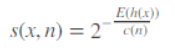树的平均路径长度为c(n)：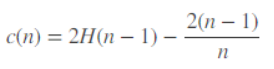H(i)为调和数，可以估计为 ln(i) + 0.5772156649。c(n)为给定样本数为 n 时，路径长度的平均值，用来标准化样本 x 的路径长度h(x)，E(h(x))为样本x在一批孤立树中的路径长度的期望。

1. 如果分数越接近1，其是异常点的可能性越高；
2. 如果分数都比0.5要小，那么基本可以确定为正常数据；
3. 如果所有分数都在0.5附近，那么数据不包含明显的异常样本。

训练：从全量数据中抽取样本，随机选择一个特征作为起始节点，并在该特征的最大值和最小值之间随机选择一个值，将样本中小于该取值的数据划到左分支，大于等于该取值的划到右分支。然后，在左右两个分支数据中重复上述步骤，直到满足如下条件：

· 数据不可再分，即：只包含一条数据，或者全部数据相同。

· 二叉树达到限定的最大深度。

预测：计算数据 x 的异常分值，先估算其在每棵树中的路径长度(即深度)。先沿着一棵树从根节点按不同特征的取值从上往下，直到到达某叶子节点。

样本点x的路径长度h(x)为从树的根节点到叶子节点所经过的边的数量。

如包含n个样本的数据集，树的平均路径长度如下：

C(s) = 2 * H(n - 1) - 2 * (n - 1) / n；H(n-1) 可用 ln(n-1) + 0.5772156649 估算。

c(n)为给定样本数为n时，路径长度的平均值，用来标准化样本 x 的路径长度h(x)。

x的异常得分：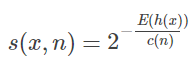从异常分值的公式看，如果数据 x 在多棵树的平均路径长度越短，得分越接近 1，表明 x 越异常；如果数据 x 的平均路径长度越长，得分越接近 0，表示数据 x 越正常；如果 x 在多棵树中的平均路径长度接近整体均值，则打分会在 0.5 附近。

实际应用：

Isolation Forest 有两个参数：二叉树的个数和训练单棵树时抽取样本的数目。

实验表明，当设定为 100 棵树，抽样样本数为 256 条时候，就可以取得不错的效果。

Isolation Forest 是无监督的异常检测算法，不需要黑白标签。

需要注意的是：

(1)如果训练样本中异常样本的比例比较高，违背了异常检测的假设，最终效果会受影响；

(2)异常检测跟具体的应用场景紧密相关，算法检测出的“异常”不一定是实际想要的。比如，在识别虚假交易时，异常的交易未必就是虚假的交易；所有在特征选择时需要过滤不太相关的特征，以免识别出一些不太相关的“异常”。

参考：

sklearn.ensemble.IsolationForest — scikit-learn 1.1.dev0 documentation

展开全文机器学习
• 1、摘要 本文主要讲解：使用孤立森林（IsolationForest）算法对 主要思路： 2、数据介绍 3、相关技术 4、完整代码和步骤 代码输出如下： 主运行程序入口 在这里插入代码片 5、学习链接

### 1、摘要

本文主要讲解：使用孤立森林（IsolationForest）算法对数据进行异常检测
主要思路：

1. 对数据进行处理，处理成算法能识别的二维数据
2. 使用孤立森林（IsolationForest）算法对数据进行聚类，分为两类，异常与正常
3. 生成网格点坐标矩阵
4. 填充等高线轮廓
5. 多种异常检测算法比较
6. 三维聚类实现

列名如下：

### 3、相关技术

在孤立森林(iForest)中，异常被定义为“容易被孤立的离群点 (more likely to be separated)”，可以将其理解为分布稀疏且离密度高的群体较远的点。 在特征空间里，分布稀疏的区域表示事件发生在该区域的概率很低，因而可以认为落在这些区域里的数据是异常的。孤立森林是一种适用于连续数据(Continuous numerical data)的无监督异常检测方法，即不需要有标记的样本来训练，但特征需要是连续的。对于如何查找哪些点容易被孤立(isolated)，iForest使用了一套非常高效的策略。在孤立森林中，递归地随机分割数据集，直到所有的样本点都是孤立的。在这种随机分割的策略下，异常点通常具有较短的路径。

直观上来讲，那些密度很高的簇是需要被切很多次才能被孤立，但是那些密度很低的点很容易就可以被孤立。
————————————————
版权声明：本文为CSDN博主「extremebingo」的原创文章，遵循CC 4.0 BY-SA版权协议，转载请附上原文出处链接及本声明。
原文链接：孤立森林（IsolationForest）算法

### 4、完整代码和步骤

代码输出如下：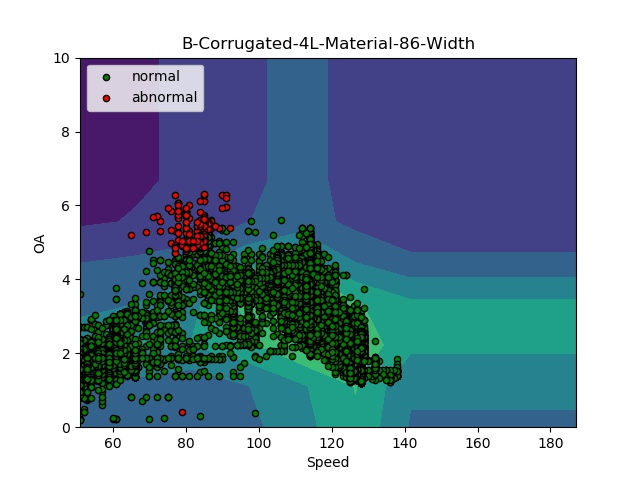# _*_coding:utf-8_*_
import numpy as np
import matplotlib.pyplot as plt
from sklearn.ensemble import IsolationForest
import pandas as pd

src1 = r'D:\data\\'
src = r'D:\data\\异常检测\\'
rng = np.random.RandomState(42)

def Generate_train_data(name, group):
float_data = group
# fit the model
clf = IsolationForest(max_samples=100,
random_state=rng, contamination=0.01)
clf.fit(float_data.values)
y_pred = clf.predict(float_data.values)
float_data.loc[:, 'category'] = y_pred.tolist()
inlier = float_data[float_data['category'] == 1][['车速']].values
outliers = float_data[float_data['category'] == -1][['车速']].values
# 生成网格点坐标矩阵
xx, yy = np.meshgrid(np.linspace(51, 187, 10), np.linspace(0, 10, 10))
x1 = xx.ravel()
# np.c_是按行连接两个矩阵，就是把两矩阵左右相加，要求行数相等
pre_z = np.c_[x1, yy.ravel()]
Z = clf.decision_function(pre_z)
Z = Z.reshape(xx.shape)
title = name + '-Corrugated' + '-' + name + '-Material-' + str(name) + '-Width'
plt.title(title)
# contourf()会填充等高线轮廓
plt.contourf(xx, yy, Z, camp=plt.cm.colors)
b1 = plt.scatter(inlier[:, 0], inlier[:, 1], c='green',
s=20, edgecolor='k')
c = plt.scatter(outliers[:, 0], outliers[:, 1], c='red',
s=20, edgecolor='k')
plt.axis('tight')
plt.xlim((51, 187))
plt.ylim((0, 10))
plt.xlabel('Speed')  # 设置x，y轴的标签
plt.ylabel('OA')
plt.legend([b1, c],
["normal", "abnormal"],
loc="upper left")

plt.show()

for name, group in groups:
print(name)
shape = group.shape
print(shape)
if shape > 110:
Generate_train_data(name, group)
else:
print(0)


三维聚类、效果不是很明显，如需直观的图请用pyecharts画

# _*_coding:utf-8_*_
import numpy as np
import matplotlib.pyplot as plt
from matplotlib import cm
from mpl_toolkits.mplot3d import axes3d
from sklearn.ensemble import IsolationForest
import pandas as pd

src1 = r'D:\data\\'

rng = np.random.RandomState(42)

def Generate_train_data(name, group):
float_data = group
# fit the model
clf = IsolationForest(max_samples=100,
random_state=rng, contamination=0.05)
clf.fit(float_data.values)
y_pred = clf.predict(float_data.values)
float_data.loc[:, 'category'] = y_pred.tolist()
inlier = float_data[float_data['category'] == 1][['车速']].values
outliers = float_data[float_data['category'] == -1][['车速']].values
# 生成网格点坐标矩阵
xx, yy = np.meshgrid(np.linspace(51, 187, 10), np.linspace(0, 12, 10))
x1 = xx.ravel()
# np.c_是按行连接两个矩阵，就是把两矩阵左右相加，要求行数相等
pre_z = np.c_[x1, yy.ravel(), yy.ravel()]
Z = clf.decision_function(pre_z)
Z = Z.reshape(xx.shape)
# z = x ** 2 + y ** 2
title = name + '-' + name + '-' + str(name)
fig = plt.figure()
ax = fig.gca(projection='3d')
# X, Y, Z = axes3d.get_test_data(0.05)
b1 = ax.scatter(inlier[:, 0], inlier[:, 1], inlier[:, 2], c='green',
edgecolor='face')
c = ax.scatter(outliers[:, 0], outliers[:, 1], outliers[:, 2], c='red',
edgecolor='face')
ax.plot_surface(xx, yy, Z, rstride=8, cstride=8, alpha=0.3)
# 绘制等高线
ax.contourf(xx, yy, Z, zdir='x', offset=187)
ax.contourf(xx, yy, Z, zdir='y', offset=12)
ax.contourf(xx, yy, Z, zdir='z', offset=12)

ax.set_xlabel('X')
ax.set_xlim(51, 187)
ax.set_ylabel('Y')
ax.set_ylim(0, 12)
ax.set_zlabel('Z')
ax.set_zlim(0, 12)

plt.title(title)
# contourf()会填充等高线轮廓
plt.axis('tight')
plt.legend([b1, c],
["inliers", "outliers"],
loc="upper left")
plt.show()

for name, group in groups:
print(name)
shape = group.shape
print(shape)
if shape > 110:
Generate_train_data(name, group)
else:
print(0)


使用孤立森林、支持向量机（SVM）、Robust 协方差估计、局部异常因子算法 Local Outlier Factor（LOF）分别对异常进行检测，并比较各自所画时间和效果

import time

import matplotlib
import matplotlib.pyplot as plt
import numpy as np
from sklearn import svm
from sklearn.covariance import EllipticEnvelope
from sklearn.ensemble import IsolationForest
from sklearn.neighbors import LocalOutlierFactor
import pandas as pd
matplotlib.rcParams['contour.negative_linestyle'] = 'solid'
src1 = r'D:\data\xiexu\\'

rng = np.random.RandomState(42)

# Example settings
n_samples = 300
outliers_fraction = 0.15
n_outliers = int(outliers_fraction * n_samples)
n_inliers = n_samples - n_outliers

# define outlier/ anomaly detection methods to be compared
anomaly_algorithms = [
("Robust covariance", EllipticEnvelope(contamination=outliers_fraction)),
("One-Class SVM", svm.OneClassSVM(nu=outliers_fraction, kernel='rbf', gamma=0.1)),
("Isolation Forest", IsolationForest(contamination=outliers_fraction, random_state=42)),
("Local Outlier Factor", LocalOutlierFactor(n_neighbors=35, contamination=outliers_fraction))
]

def Generate_train_data(group):
# define datasets
float_data = group.values
X_train = float_data[:300]
# X_test = float_data[300:]
datasets = [X_train]

xx, yy = np.meshgrid(np.linspace(51, 187, 150), np.linspace(0, 12, 150))

plt.figure(figsize=(len(anomaly_algorithms) * 2 + 3, 12.5))
plt.subplots_adjust(left=0.02, right=0.98, bottom=0.001, top=0.96, wspace=0.05, hspace=0.01)

# rng = np.random.RandomState(42)
plot_num = 1
for i_dataset, X in enumerate(datasets):
# outliers = rng.uniform(low=-6, high=6, size=(n_outliers, 2))
# X = np.concatenate([X, outliers], axis=0)
for name, algorithm in anomaly_algorithms:
print(name, algorithm)
t0 = time.time()
algorithm.fit(X)
t1 = time.time()
plt.subplot(2, 2, plot_num)
if i_dataset == 0:
plt.title(name, size=18)

# fit the data and tag outliers
if name == 'Local Outlier Factor':
y_pred = algorithm.fit_predict(X)
else:
y_pred = algorithm.fit(X).predict(X)

# plot the levels lines and the points
if name != "Local Outlier Factor":
Z = algorithm.predict(np.c_[xx.ravel(), yy.ravel()])
Z = Z.reshape(xx.shape)
plt.contour(xx, yy, Z, levels=, linewidths=2, colors='black')

colors = np.array(["#377eb8", '#ff7f00'])
plt.scatter(X[:, 0], X[:, 1], s=10, color=colors[(y_pred + 1) // 2])

plt.xlim(51, 187)
plt.ylim(0, 12)
plt.xticks(())
plt.yticks(())
plt.text(0.99, 0.01, ('%.2fs' % (t1 - t0)).lstrip('0'),
transform=plt.gca().transAxes, size=15,
horizontalalignment='right')
plot_num += 1

plt.show()

for name, group in groups:
print(name)
if group.shape > 300:
Generate_train_data(group)
else:
print(0)


### 5、学习链接

sklearn四种异常检测算法比较以及实现异常点检测

展开全文聚类 算法 机器学习 SVM
• 孤立森林（Isolation Forest） 怎么来切这个数据空间是iForest的设计核心思想 思路： 将整个数据划分为多个子集，在子集上进行异常检测的操作。iTree的建立是通过对训练集的递归分隔来建立的，直到所有的样本被孤立...

# 孤立森林（Isolation Forest）

怎么来切这个数据空间是iForest的设计核心思想

## 思路：

将整个数据划分为多个子集，在子集上进行异常检测的操作。iTree的建立是通过对训练集的递归分隔来建立的，直到所有的样本被孤立，或者树达到了指定的高度，树的高度限制l与子样本数量ψ的关系为l=ceiling(log2(ψ))，它近似等于树的平均高度。我们只关心路径长度较小的那些点，它们更有可能是异常点，而并不关心路径很长的正常点。获得t个iTree之后，iForest 训练就结束，然后我们可以用生成的iForest来评估测试数据了。对于一个训练数据x，我们令其遍历每一棵iTree，然后计算x最终落在每个树第几层（x在树的高度）。是另外一种高效的异常检测算法，它和随机森林类似，但每次选择划分属性和划分点（值）时都是随机的（每次节点都会随机选取一个特征），而不是根据信息增益或者基尼指数来选择。在建树过程中，如果一些样本很快就到达了叶子节点（即叶子到根的距离d很短），那么就被认为很有可能是异常点。因为那些路径d比较短的样本，都是因为距离主要的样本点分布中心比较远的。也就是说，可以通过计算样本在所有树中的平均路径长度来寻找异常点。在一棵树的根节点选取的属性为A，那么在根节点的子节点上选取的属性还是A吗？可能是A也可能是别的属性。

## 优点

对故障诊断来说，孤立森林所拥有的优点。
1.适用于连续数据，无监督学习，无需对未打标签的数据进行标记即可训练。

## 缺点

对故障诊断来说，孤立森林所拥有的缺点。
1.样本数较多会降低孤立森林孤立异常点的能力，因为正常样本会干扰隔离的过，降低隔离异常的能力。子采样就是在这种情况下被提出的。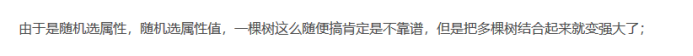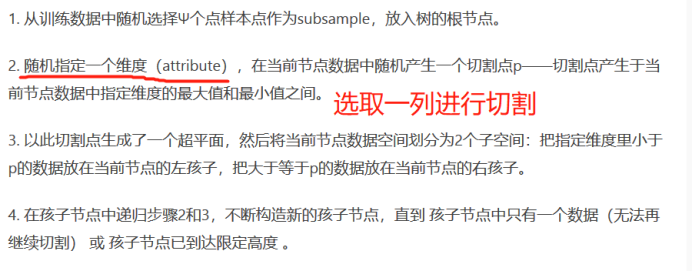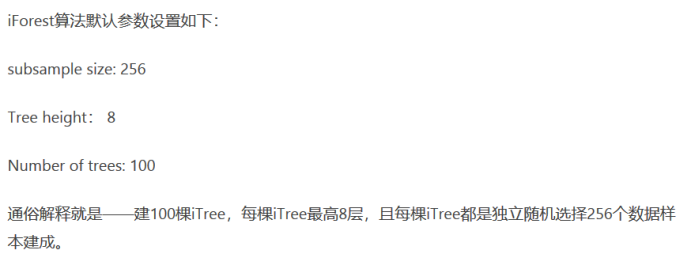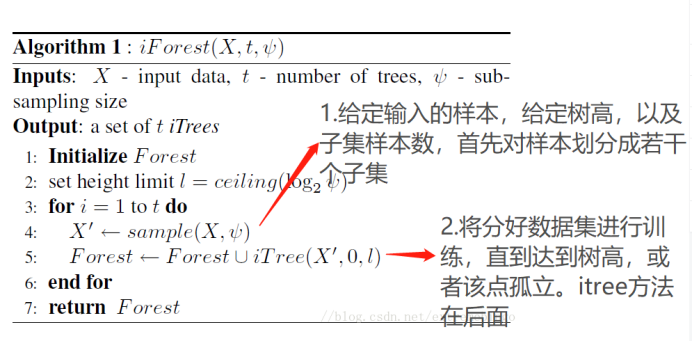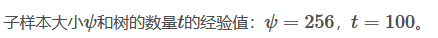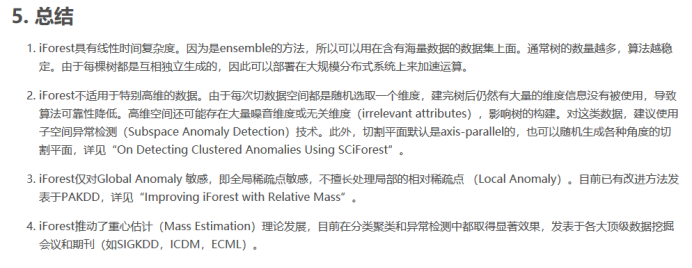## 代码

import numpy as np
import matplotlib.pyplot as plt
from sklearn.ensemble import IsolationForest
rng=np.random.RandomState(42)
# 生成训练数据
X=0.3*rng.randn(100,2) # 100条二维数据
X_train=np.r_[X+2,X-2] # 200条数据（X+2,X-2）拼接而成

X = 0.3 * rng.randn(20, 2)
X_test = np.r_[X + 2, X - 2]
# 基于分布生成一些观测正常的数据
X_outliers=rng.uniform(low=-4,high=4,size=(20,2))
# 训练隔离森林模型
clf=IsolationForest(behaviour='new',max_samples=100,random_state=rng,contamination='auto')
clf.fit(X_train)
y_pred_train=clf.predict(X_train)
y_pred_test=clf.predict(X_test)
y_pred_outliers = clf.predict(X_outliers)
# 画图
xx, yy = np.meshgrid(np.linspace(-5, 5, 50), np.linspace(-5, 5, 50)) # 生成网络数据 https://www.cnblogs.com/lemonbit/p/7593898.html
Z = clf.decision_function(np.c_[xx.ravel(), yy.ravel()])
Z = Z.reshape(xx.shape)

plt.title("IsolationForest")
plt.contourf(xx, yy, Z, cmap=plt.cm.Blues_r) # 等高线

b1 = plt.scatter(X_train[:, 0], X_train[:, 1], c='white',
s=20, edgecolor='k')
b2 = plt.scatter(X_test[:, 0], X_test[:, 1], c='green',
s=20, edgecolor='k')
c = plt.scatter(X_outliers[:, 0], X_outliers[:, 1], c='red',
s=20, edgecolor='k')
plt.axis('tight')
plt.xlim((-5, 5))
plt.ylim((-5, 5))
plt.legend([b1, b2, c],
["training observations",
"new regular observations", "new abnormal observations"],
loc="upper left")
plt.show()


## 参考博客：

https://blog.csdn.net/u013709270/article/details/73436588
https://blog.csdn.net/extremebingo/article/details/80108247
https://www.jianshu.com/p/5af3c66e0410?utm_campaign=maleskine
https://www.cnblogs.com/wanghui-garcia/p/11475713.html程序
https://zhuanlan.zhihu.com/p/52282709
https://blog.csdn.net/ye1215172385/article/details/79762317 主要参数
https://blog.csdn.net/weixin_30905133/article/details/101564568 主要参数
https://www.jianshu.com/p/e408a010e424有注释的代码

展开全文• 孤立森林训练 def score(filename2,filename3): dataset = pd.read_csv(filename2, engine='python') dataset = dataset.fillna(0) X_col = pd.DataFrame(dataset, columns=['avg_velocity_qian','avg_velocity','...
• 第二部分介绍孤立森林算法（iForest），Isolation Forest 简称 iForest，该算法是周志华在2010年提出的一个异常值检测算法，在工业界很实用，算法效果好，时间效率高。第二部分包括对iForest算法思想、原理、流程的...算法 机器学习 人工智能
• 目录什么是Isolation Tree和Isolation Forest...test, F-test)来发现异常点，Isolation Tree巧妙的利用决策树分裂来寻找离群样本，由于异常点和大多数的正常样本差距较大所以会更早的被孤立出来，如下图所示：标红...
• 孤立森林(Isolation Forest)简称iForest，是无监督的模型，常用于异常检测。在一大堆数据中，找出与其它数据的规律不太符合的数据 孤立森林将异常识别为树上平均路径较短的观测结果。每个孤立树都应用了一个过程: ...矩阵 python 机器学习
• 孤立森林是基于Ensemble的快速异常检测方法，具有线性时间复杂度和高精准度，是符合大数据处理要求的state-of-the-art算法。孤立森林算法适用于连续数据的异常检测，将异常定义为“容易被孤立的离群点”，可以理解...python
• 本发明涉及异常检测技术领域，尤其是一种将孤立森林算法模块化与可视化的方法。背景技术：异常检测算法已有常用的几个流行算法，如LOF算法、COF算法和DBSCAN算法，只是算法模块化和可视化的方法并没有出现。随着数据...
• 最近正好在做孤立森林的项目，写下这篇推文记录下项目进程，也给需要做这个项目的同学一点参考。    1. 什么叫异常点   图中红色的点偏离大部分的数据，可以发现这些点分布稀疏且离密度高的群体较远，这样的点...python
• 1背景 现有的异常检测方法主要是通过对正常样本的描述，给出一个正常样本在特征空间中的区域，... 孤立森林，不再是描述正常的样本点，而是要孤立异常点，由周志华教授等人于2008年在第八届IEEE数据挖掘国际会议上...算法 机器学习 人工智能
• 这类的数据被称为异常值，需要被试别和区分。造成这些异常现象的原因有很多:数据的可变性、数据收集过程中获得的错误，或者发生了一些新的、罕见的情况。 管理这些离群值很有挑战性的，因为通常不可能理解问题是否与...
• 孤立森林或“iForest”是一个非常漂亮和优雅简单的算法，可以用很少的参数来识别异常。原始的论文对广大的读者来说是容易理解的，并且包含了很少的数学知识。在这篇文章中，我将解释为什么iForest是目前最好的大数据...python 大数据 决策树 异常检测
• 孤立森林(Isolation Forest)简称iForest，此算法对内存要求很低，且处理速度很快，其时间复杂度也是线性的。可以很好的处理高维数据和大数据，并且也可以作为在线异常检测。 算法简介      ...
• 孤立森林Isolation Forest(sklearn.ensemble.IsolationForest)：一种适用于连续数据的无监督异常检测方法。与随机森林类似，都是高效的集成算法，相较于LOF，K-means等传统算法，该算法鲁棒性高且对数据集的分布无...
• 基于时间序列分析 同比环比 基于统计 单特征且符合正态分布 基于统计 箱线图 基于聚类 DBSCAN 基于树模型 孤立森林 基于线性模型 OneClassSVM 基于密度 LOF机器学习 异常检测
• 01 孤立森林 isolation，意为孤立/隔离，是名词，其动词为isolate，forest是森林，合起来就是“孤立森林”了，也有叫“独异森林”，好像并没有统一的中文叫法。可能大家更习惯用其英文的名字isolation forest，简称...机器学习 异常检测 数据挖掘
• contamination=’legacy’, max_features=1.0, bootstrap=False, n_jobs=None, behaviour=’old’, random_state=None, verbose=0, warm_start=False) 孤立森林算法 使用孤立森林算法对每个样本返回异常分数 孤立...
• label, columns=['outlier_label']) # 将标签数据与原来的数据合并 data_merge = pd.concat((df, outlier_pd), axis=1) normal_source = data_merge[data_merge['outlier_label']==1] normal_source 5.测试效果 IF_...python 机器学习 开发语言
• 社群发现也叫社区发现，是用来发掘分离出网络中子网内聚较高关系密切的一组组子网结构。...在此用自动化的iForest（孤立森林）算法去除离群的低频标签，代替了人为阈值过滤方式。iForest属于Non-parametri...
• // 孤立森林 import numpy as np from sklearn.ensemble import IsolationFores import matplotlib.pyplot as plt #data前两列是数据，第三列是标签 data = np.loadtxt(open("data_919.csv","rb"),delimiter=",",...
• 高维数据中，并非所有的样本都会用到，需要特征选择； 由于样本高度不平衡，因此测试的指标往往用F1； 异常检测问题，往往更多使用无监督的算法建模，再结合标定的验证集用于切阈值。 异常检测的两类常用方法： ....异常检测
• Forest （Isolation Forest）孤立森林 是一个基于Ensemble的快速异常检测方法，具有线性时间复杂度和高精准度。其可以用于网络安全中的攻击检测，金融交易欺诈检测，疾病侦测，和噪声数据过滤等。 动机 目前...
• // 孤立森林 import numpy as np from sklearn.ensemble import IsolationFores import matplotlib.pyplot as plt #data前两列是数据，第三列是标签 data = np.loadtxt(open("data_919.csv","rb"),delimiter=",",...机器学习 python...